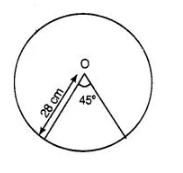# Find the area of a sector of a circle

Question:

Find the area of a sector of a circle of radius 28 cm and central angle 45°.

Solution:

Given that, Radius of a circle, r = 28 cm

and measure of central angle θ= 45°

Hence, the required area of a sector of a circle is 308 cm$\therefore \quad$ Area of a sector of a circle $=\frac{\pi r^{2}}{360^{\circ}} \times \theta$

$=\frac{22}{7} \times \frac{(28)^{2}}{360} \times 45^{\circ}$

$=\frac{22 \times 28 \times 28}{7} \times \frac{45^{\circ}}{360^{\circ}}$

$=22 \times 4 \times 28 \times \frac{1}{8}$

$=22 \times 14$

$=308 \mathrm{~cm}^{2}$